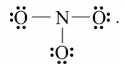The hybridization of N in  NO2+,NO3− and NH4+ respectively are

# The hybridization of N in  ${\mathrm{NO}}_{2}^{+},{\mathrm{NO}}_{3}^{-}$ and ${\mathrm{NH}}_{4}^{+}$ respectively are

1. A

$\mathrm{sp},{\mathrm{sp}}^{2},{\mathrm{sp}}^{3}$

2. B

$\mathrm{sp},{\mathrm{sp}}^{3},{\mathrm{sp}}^{2}$

3. C

${\mathrm{sp}}^{2},\mathrm{sp},{\mathrm{sp}}^{3}$

4. D

${\mathrm{sp}}^{3},{\mathrm{sp}}^{2},\mathrm{sp}$

Register to Get Free Mock Test and Study Material

+91

Live ClassesRecorded ClassesTest SeriesSelf Learning

Verify OTP Code (required)

### Solution:

${\mathrm{NO}}_{2}^{+}$ has 16 valence electrons. There arrangement will be  Two pairs of electrons around N implies linear geometry involving sp hybridization. NO3 has 24 (= 5 x 3 x 6 + 1) valence electrons. These are arranged asThere are three pairs of electrons around N. Its geometry will be trigonal planar and will result from ${\mathrm{sp}}^{2}$ hybridization. ${\mathrm{NH}}_{4}^{+}$ has  ${\mathrm{sp}}^{3}$ hybridization.